How to cite this paper

Marcoux, Yves, Michael Sperberg-McQueen and Claus Huitfeldt. “Modeling overlapping structures: Graphs and serializability.” Presented at Balisage: The Markup Conference 2013, Montréal, Canada, August 6 - 9, 2013. In Proceedings of Balisage: The Markup Conference 2013. Balisage Series on Markup Technologies, vol. 10 (2013). https://doi.org/10.4242/BalisageVol10.Marcoux01.

Balisage: The Markup Conference 2013
August 6 - 9, 2013

# Balisage Paper: Modeling overlapping structures

## Graphs and serializability

Yves Marcoux

Associate professor

Université de Montréal, Canada

##### `<[email protected]>`

Michael Sperberg-McQueen

Senior consultant

Black Mesa Technologies

##### `<[email protected]>`

Claus Huitfeldt

Associate professor

University of Bergen, Norway

##### `<[email protected]>`

Copyright © 2013 by the authors. Used with permission.

## Abstract

The problem of overlapping structures has long been familiar to the structured document community. In a poem, for example, the verse and line structures overlap, and having them both available simultaneously is convenient, and sometimes necessary (for example for automatic analyses). However, only structures that embed nicely can be represented directly in XML. Proposals to address this problem include XML solutions (based essentially on a layer of semantics) and non-XML ones. Among the latter is TexMecs HS2003, a markup language that allows overlap (and many other features).

XML documents, when viewed as graphs, correspond to trees. Marcoux M2008 characterized overlap-only TexMecs documents by showing that they correspond exactly to completion-acyclic node-ordered directed acyclic graphs. In this paper, we elaborate on that result in two ways.

First, we cast it in the setting of a strictly larger class of graphs, child-arc-ordered directed graphs, that includes multi-graphs and non-acyclic graphs, and show that — somewhat surprisingly — it does not hold in general for graphs with multiple roots. Second, we formulate a stronger condition, full-completion-acyclicity, that guarantees correspondence with an overlap-only document, even for graphs that have multiple roots.

The definition of fully-completion-acyclic graph does not in itself suggest an efficient algorithm for checking the condition, nor for computing a corresponding overlap-only document when the condition is satisfied. We present basic polynomial-time upper bounds on the complexity of accomplishing those tasks.

## Table of Contents

1. Motivation and related work
1.1. Graphs and documents
1.2. The problem of serialization
1.3 The approach of this paper
2. Child-arc-ordered directed graphs
3. Overlap-only documents
4. Correspondence between a graph and a document
5. Main results
6. Checking full-completion-acyclicity
7. Conclusion and future work
Appendix A. Notation and symbols

## 1. Motivation and related work

### 1.1. Graphs and documents

Many operations are more conveniently performed on a graph representation than on a linear representation of a marked up document, and vice versa. One of the strengths of XML is that XML documents in serial form are readily deserialized into ordered trees, which form a convenient data structure for many useful operations.

So-called “XML trees” are directed acyclic graphs with single parenthood and a total ordering on leaf nodes. While this constitutes an intuitively natural and generally suitable model for the representation of the structure of most documents, and for most purposes, it also poses a challenge for the representation of complex structures such as overlapping, fragmented or disordered document elements, and multiple co-existing alternative structures, which allow for a more natural representation of complex documents in a wide range of situations.

For such purposes, a different kind of graph representation has been proposed, the so-called Goddag SH2004. Roughly, Goddags (General Ordered-Descendant Directed Acyclic Graphs) are like XML trees except that they allow multiple parenthood and do not require a total ordering on leaf nodes. (Thus, XML trees constitute a subset of Goddags.)

Documents using different techniques for representing such structures in XML form (e.g., milestones, fragmentation, virtual elements, etc. B1995 SH1999 W2005) can be mapped onto Goddags, though not without application-specific mechanisms typically involving levels of indirection which may appear cumbersome. The experimental markup system TexMecs HS2003 offers mechanisms for the representation of complex structures which can be mapped on to Goddags independently of such knowledge.

Since its introduction, the Goddag data structure has frequently been cited, and it is used as a reference in various works on overlap. (For example, Moore M2012 studied Goddags in the context of access control, and introduced the notion of globally ordered Goddag.) However, the original description of Goddags is rather informal, and exhibits the kinds of gaps, vaguenesses, and ambiguities that have, over time, given informality a bad name among mathematicians and others interested in firm results.

For example, it was conjectured that a linearized document which made use, in addition to the mechanisms of XML, only of markup for overlapping elements, could be represented by a Goddag with a total order on leaf nodes (so-called restricted Goddags), but no proofs were given of this fact. The paper was silent and its authors agnostic about the serializability of graphs with multiple roots, and the relationship between Goddags and markup in terms of serializability was not systematically investigated. In HS2003, it was assumed, but no attempt was made to prove, that all TexMecs documents could be represented as Goddags, or that all Goddags could be serialized as TexMecs documents. The present paper is a modest contribution towards straightening up the situation, by way of a systematic study of the fine point of the complex relationship between markup formalisms like TexMecs and graph structures like Goddags.

### 1.2. The problem of serialization

The general problem is this: whenever a markup system (be it XML, TexMecs, or another system) provides more than one way to represent a given abstract structure, that same abstract structure can be written out again (serialized) in more than one way. Can we control the serialization process to provide the marked-up forms we find easiest to work with at a given moment? Can we tell, by inspection of a given graph, what serialization formats are possible for the graph? In many cases, a marked up form using overlapping elements, seems to at least some observers to be the most natural representation of a given document; when can a graph be serialized using overlap alone, and when does it require use of the more powerful mechanisms of virtual or discontinuous elements?

A concrete example may help illustrate the point.

In the following fragment (adapted from D2004), the `verse` elements are empty milestones marking the beginning and end of verses, in Trojan Horse style markup. A Goddag structure representing this fragment would have nodes for the verses, but those nodes do not correspond one to one with XML elements in this serialization:

```<div xmlns="http://www.tei-c.org/ns/1.0">
<p>
<verse xml:id="Jer.2.1"/>
Moreover the word of the LORD
came to me, saying,
<verse eID="#Jer.2.1"/>
<q n="Q-Jer.2.2-A">
<verse xml:id="Jer.2.2"/>
Go and cry in the
hearing of Jerusalem, saying,
<q n="Q-Jer.2.2-B">
Thus says the LORD:
<q n="Q-Jer.2.2-C">
I remember you,
The kindness of your youth,
The love of your betrothal,
When you went after Me in the wilderness,
In a land not sown.
<verse eID="#Jer.2.2"/>
<verse xml:id="Jer.2.3"/>
Israel [was] holiness to the LORD,
The firstfruits of His increase.
All that devour him will offend;
Disaster will come upon them,
</q>
<!--True Close Q-Jer.2.2-C-->
says the LORD.</q>
<verse eID="#Jer.2.3"/>
<!--* ... *-->
</q>
</p>
</div>```

The same Goddag structure can also be serialized in an extended form of TexMecs notation.

```<div|
<p|
<[email protected]|Moreover the word
of the LORD came to me, saying,|verse>
<q n="Q-Jer.2.2-A"|
<[email protected]|
Go and cry in the
hearing of Jerusalem, saying,
<[email protected]|
Thus says the LORD:|~>
<[email protected]|
I remember you,
The kindness of your youth,
The love of your betrothal,
When you went after Me in the wilderness,
In a land not sown.|~>
|verse>
<q n="Q-Jer.2.2-B"|
<[email protected]=>
<q n="Q-Jer.2.2-C"|
<=Jer.2.2c=>
<[email protected]|
Israel [was] holiness to the LORD,
The firstfruits of His increase.
All that devour him will offend;
Disaster will come upon them,
|~>
|q>
|q>
<[email protected]|
<=Jer.2.3a=>
says the LORD.
|verse>
<* ... *>
|q>
|p>
|div>```

This particular Goddag structure can also be serialized without virtual elements, just by allowing the `q` and `verse` elements to overlap:

```<div|
<p|
<[email protected]|
Moreover the word of the LORD
came to me, saying,
|verse>
<q n="Q-Jer.2.2-A"|
<[email protected]|
Go and cry in the
hearing of Jerusalem, saying,
<q n="Q-Jer.2.2-B"|
Thus says the LORD:
<q n="Q-Jer.2.2-C"|
I remember you,
The kindness of your youth,
The love of your betrothal,
When you went after Me in the wilderness,
In a land not sown.
|verse>
<[email protected]|
Israel [was] holiness to the LORD,
The firstfruits of His increase.
All that devour him will offend;
Disaster will come upon them,
|q>
says the LORD.
|verse>
|q>
<* ... etc. ... *>
|q>
|p>
|div>```
Intuitively, many readers find the overlap-only version of the document simpler and more natural than the version using bilocation tags. But (as demonstrated by M2008), not all Goddag structures can be written out using only overlap, without virtual elements, discontinuous elements, or bilocation tags.

This leads directly and obviously to the questions When can graphs be serialized using overlap only? And conversely, when are other markup mechanisms necessary?

Marcoux M2008 introduced the notions of noDAG and overlap-only (oo) TexMecs as a first step towards answering these questions. A noDAG is a node-ordered directed acyclic graph, i.e., a slight variation on the Goddag, where there is a strict partial ordering on nodes. As a markup language, oo-TexMecs is the subset of TexMecs that allows multiple roots and overlapping elements, but not virtual or interrupted elements. Marcoux established that a noDAG is serializable if and only if it is completion-acyclic, and that “round-tripping” is possible, in that there is essentially a bijective correspondence between noDAGs and oo-TexMecs documents.

### 1.3 The approach of this paper

In order to investigate whether and how the results of M2008 apply to other classes of graphs, we introduce here the more general notion of a child-arc-ordered directed graph (CODG), and demonstrate that the results from M2008 hold also for CODGs, with the somewhat surprising exception of some CODGs with multiple roots. By defining the stronger notion of “fully completion-acyclic” graphs, we succeed in identifying this subset: the oo-serializable CODGs are exactly the fully-completion-acyclic ones. We also give basic polynomial-time upper bounds on the complexity of checking full-completion-acyclicity and of actually computing an oo-serialization of fully-completion-acyclic CODGs.

## 2. Child-arc-ordered directed graphs

2.1 Definition A child-arc-ordered directed graph (CODG for short) G = (V, ch) is a directed graph over a finite non-empty set of vertices (or nodes) V, where ch (for children) is a total mapping from V to finite (and possibly empty) sequences of nodes from V. The set of arcs (or edges) of G, noted E(G), comprises exactly those ordered pairs (v, w) for which

(∃n ∈ N)[ ch(v, n) = w ],

where N represents the set of non-negative integers. The notation ch(v, n) is used as a shorthand for (ch(v))(n), that is, for the element with index n in the sequence ch(v). We use 0-origin indexing; thus, for all v ∈ V, ch(v, 0) denotes the first child of v (or is undefined, if v has no child).

Note: Throughout this paper, the “parent” relation must be understood to be the exact inverse of the “child” relation (we bother to make this explicit because it is not the case in some other models, such as the XPath 1.0 data model).

It is possible for the same child to show up at more than one place in a sequence of children; that is, ch(v, n) = ch(v, m) with m ≠ n is possible. Loops are allowed; that is, ch(v, n) = v for a given n is possible.

Note that (v, w) ∈ E(G) for given v and w tells only part of the story: There could be many distinct values of n for which ch(v, n) = w. Also note that the length of ch(v), i.e., the smallest value of n (≥ 0) for which ch(v, n) is undefined, is greater than or equal to the number of distinct children of v (if v has no child, ch(v) = ∅, which, as a sequence, is of length 0).

CODGs are very loose structures: they can be “multi-graphs,” in that more than one arc can link any given pair of nodes. They can have cycles and loops (i.e., cycles of length one). There can be both a direct (length one) and indirect path between any two given nodes.

The rationale for the adjective child-arc-ordered is that for all v ∈ V, ch(v) can be seen as inducing an ordering on the arcs going out of v (the child-arcs of v).

Examples We present examples of CODGs illustrating some of their features.Example 2.1

Example 2.1 illustrates that CODGs can be disconnected, and that, in a CODG:

• A node can have more than one parent (here most simply node d, with parents a and c, but also node b [with parents a and b]). This is a significant departure from the rule of single parenthood in XML.

• There can be cycles and loops (cycles of length 1); here the only example is the loop on node b.

• The same node can occur more than once as a child of some parent (here node c, which is both second and fourth among the children of node a).

• There can be both direct and indirect paths between two nodes (here node a dominates node d both directly and via node c).

Note that the order of the outgoing arcs is usually not shown explicitly in the visual representation of a CODG. We adopt the convention of drawing the arcs going out of any node in order from left to right (even if the arcs must cross each other further down, in order to reach the child node they point to). So the leftmost arc leaving any parent is pointing to that parent's first child, and the rightmost arc points to that parent's last child. Thus, in Example 1, ch(a) = (b, c, d, c).

On the rare occasions that this convention is not practical, we use explicit green arrows between the outgoing arcs to indicate their order, as in the next example.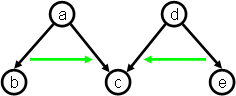Example 2.2

In Example 2.2, ch(a) = (b, c) and ch(d) = (e, c). Right-pointing arrows, though superfluous, are sometimes shown as a reminder of the implicit convention.

Sibling precedence For all v, ch(v) induces a sibling-precedence relation sp(v) among the children of v, defined by:

sp(v) =def { (w, x) ∈ V × V | (∃m, n ∈ N)[ m < n & ch(v, m) = w & ch(v, n) = x ] }.

This relation may or may not be a strict order relation. When it is, we say that v orders its children.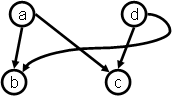Example 2.3

Example 2.3 illustrates that parents may order the same nodes differently as children. Thus, note that ch(a) = (b, c), which induces the strict order relation b < c, and ch(d) = (c, b), which induces the strict order relation c < b.

In examples in which no pair of nodes is ordered differently by different parents, we will usually place the green arrows between nodes, rather than between arcs: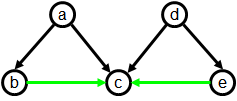Example 2.4

Auxiliary concepts We now define a number of auxiliary concepts useful in discussions of CODGs. All of them are secondary concepts in the sense that they are entirely and uniquely determined by the set of vertices and the sequences of children of the graph.

2.2 Definition Let G = (V, ch) be a CODG. Then:

1. G denotes the (positive) transitive closure of E(G).

Note that ⇒G is not necessarily antireflexive, as E(G) may contain cycles.

2. *G denotes the reflexive transitive closure of E(G), that is:

G ∪ { (v, v) | v ∈ V }.

3. sp(G) =def { (v, w, x) ∈ V × V × V | (∃m, n ∈ N)[ m < n & ch(v, m) = w & ch(v, n) = x ] }.

The name “sp” stands for “sibling precedence.” Note that, iff w occurs more than once in the sequence of children of v, then (v, w, w) ∈ sp(G). Note also that it is entirely possible for both (v, w, x) and and (v, x, w) to be members of sp(G). Finally note that sp(G) is the union over all v ∈ V of ({v} × sp(v)), where sp(v) is the sibling-precedence relation induced by ch(v), as defined above at Sibling precedence.

4. gsp(G) =def { (w, x) ∈ V × V | (∃v ∈ V)[ (v, w, x) ∈ sp(G) ] }.

The name “gsp” stands for “global sibling precedence.” It is the projection of sp(G) onto the last two components. It follows from the observations in the preceding point that there can be loops and cycles in gsp(G).

2.3 Notation Let G = (V, ch) be a CODG. Unless otherwise stated:

1. V can also be denoted by V(G),

2. E denotes E(G),

3. ⇒ denotes ⇒G,

4. * denotes ⇒*G,

5. sp denotes sp(G),

6. gsp denotes gsp(G).

## 3. Overlap-only documents

The phenomenon we wish to study in this paper is how the structural properties of a CODG relate to the fact that it mimics the containment and precedence relationships among elements in some marked-up document. More specifically, we want to consider documents expressed in markup languages that allow overlapping elements, such as TexMecs HS2003. Thus, we need to define a model for such documents.

TexMecs allows many more constructs than element embedding and overlap. However, in this paper, we concentrate on those two, ignoring the others, such as virtual elements, interrupted elements, empty elements, attribute specifications, entity references, generic identifier co-indexing (for handling self-overlap), unordered contents, and comments. This is why we speak of “overlap-only” (or “oo”) documents. When the structure of a CODG corresponds to the containment and precedence relationships of some oo-document (to be defined precisely in a moment), we say the CODG is “oo-serializable,” because the oo-document can be viewed as a serialization (a representation in serial form) of the CODG.

Instead of defining documents as character strings with syntactic constraints, we use a more abstract approach that avoids some complications and leads to results that are simpler to formulate. More constraints on the definition of document could later be added to suit specific markup languages if and when desired.

Intuitively, we adopt a tokenized view of the document, where tokens are tags and leaves. Tokens are represented by their (integer) position in the sequence of tokens that make up the document.

The tags in our model of oo-documents correspond, in the XML world, to start- and end-tags for non-empty elements.

The leaves in our model of oo-documents correspond, in the XML world, to text nodes (#PCDATA) and empty elements. Note that our model abstracts away from the actual textual content of elements and documents, and also ignores the differences among different element types. We claim, however, that our abstraction captures the essential structural aspects of marked-up documents with possible element overlap.

3.1 Definition An oo-document is a finite set of pairs of the form (x, y), where x, y ∈ N (the set of non-negative natural numbers) and x ≤ y, additionally satisfying a number of “well-formedness” constraints (stated below).

The pairs in a document are called ranges. If r = (x, y) is a range, then r1 and r2 denote respectively x and y.

Intuitively, a range gives the position of a start-tag and of the corresponding end-tag, or the position of a leaf, in which case, x = y. Formally, if D is an oo-document:

• leaves(D) =def { x ∈ N | (x, x) ∈ D },

• stags(D) =def domain(D) - leaves(D),

• etags(D) =def image(D) - leaves(D).

Note that, as usual:

• domain(D) =def { x ∈ N | ( ∃ y ∈ N | (x, y) ∈ D ) }, and

• image(D) =def { y ∈ N | ( ∃ x ∈ N | (x, y) ∈ D ) }, and

Oo-documents are subject to the following well-formedness constraints.

For all oo-document D:

1. D is a partial function over N, i.e., for all x ∈ N, there is at most one y such that (x, y) ∈ D.

2. D-1 (that is, the inverse of D) is also a partial function over N, i.e., for all y ∈ N, there is at most one x such that (x, y) ∈ D.

3. stags(D) ∩ etags(D) = ∅.

Put less formally: No token is both a start-tag and an end-tag.

It must also be remembered that (as stated at the beginning of the definition) for all range r, r1 ≤ r2, which corresponds to the normal rule of syntax that start-tag must precede its matching end-tag.

Note that we do not require the numbering of token positions to be gap-free, nor do we forbid consecutive leaves without intervening tags. There is also no requirement of an element spanning the whole document: this is of course crucial for oo-documents to be able to correspond to graphs with multiple roots.

3.2 Definition Let D be an oo-document, and r, s ∈ D:

• r is said to contain s iff (r1 < s1 and s2 < r2).

• r is said to precede s iff (r1 < s1 and r2 < s2).

Note that in the latter case, r and s may or may not overlap. Also note that r cannot both contain and precede s.

## 4. Correspondence between a graph and a document

Intuitively, a CODG and an oo-document correspond to each other when the nodes of the graph and the ranges of the document can be put in correspondence in such a way that node reachability corresponds to range containment, and gsp corresponds to range precedence.

4.1 Definition A CODG G and an oo-document D correspond to each other iff there exists a bijective mapping g from V(G) to D, such that all of the following conditions hold:

1. (∀ b, c ∈ V(G)) [(b ⇒ c) iff g(b) contains g(c)]

2. (∀ b, c ∈ V(G)) [if (b, c) ∈ gsp(G), then g(b) precedes g(c)]

We then say that G and D correspond to each other through g.

4.2 Definition A CODG G is said to be oo-serializable iff there exists an oo-document that corresponds to G.

It is clear that every oo-document has a corresponding CODG: use ranges as nodes, and the transitive reduction of range containment as parent-child relation. Then, order all sets of siblings in range precedence order.

It is also clear that some CODGs are not oo-serializable: for example, a CODG with cycles would imply (by transitivity) a range containing itself, which is impossible. But are all acyclic CODGs oo-serializable? The following examples, inspired from M2008, show that the question is at best not trivial: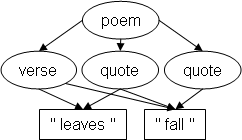Example 4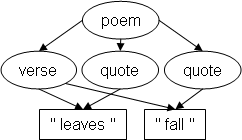Example 5

Both graphs are acyclic and they differ by just the presence/absence of one arc. Yet, only the first one is oo-serializable M2008.

In M2008, Marcoux defined noDAGs, or node-ordered DAGs, as (essentially) directed acyclic graphs (DAGs) in which the nodes are partially ordered in such a way that siblings (children of a common parent), as well as distinct roots, are totally ordered. He then defined the property of completion-acyclicity for noDAGs, and showed that oo-serializable noDAGs are exactly the completion-acyclic ones.

In order to investigate whether the same is true of CODGs, we must define an analogous property for CODGs. The following definition is the natural adaptation of completion-acyclicity to CODGs.

4.3 Definition Let G = (V, ch) be a CODG. Then:

1. ssba(G) =def { (w, x) ∈ V × V | (∃v ∈ V)[ v ⇒ w & (v, x) ∈ gsp & x ⇏ w ] }.

The name “ssba” stands for “should-start-before additions.”

2. ssb(G) denotes the transitive closure of (E ∪ gsp ∪ ssba).

The relation “ssb” is called the “should-start-before completion” of G.

3. seaa(G) =def { (w, x) ∈ V × V | (∃v ∈ V)[ v ⇒ w & (v, x) ∈ gsp-1 & x ⇏ w ] }.

The name “seaa” stands for “should-end-after additions.” The relation gsp-1 is the inverse of relation gsp.

4. sea(G) denotes the transitive closure of (E ∪ gsp-1 ∪ seaa).

The relation “sea” is called the “should-end-after completion” of G.

4.4 Notation Let G = (V, ch) be a CODG. Unless otherwise stated:

1. ssba denotes ssba(G),

2. ssb denotes ssb(G),

3. seaa denotes seaa(G),

4. sea denotes sea(G).

The relations ssb and sea can be understood as meaning: “should <something> in any oo-serialization of the CODG,” for example “should end after in any oo-serialization of the CODG.” Thus, “(v, w) ∈ ssb” can be read out as: “v should start before w in any oo-serialization of the CODG.” In other words, ssb (respectively, sea) represents the start- (respectively, end-) tag-precedence relations that can be deduced from the topology of the CODG, supposing parent-child relations are interpreted as element containment, and sibling-precedence relations as start- and end-tag-precedence.

The relations ssba and seaa represent the “additional” arcs (over and above those in E and gsp or gsp-1) that must be considered to compute all the possible ssb and sea pairs that can be deduced from the CODG topology.

4.5 Definition A CODG G = (V, ch) is said to be completion-acyclic (CA) iff each of ssb(G) and sea(G) is acyclic.

## 5. Main results

Things are not as simple with CODGs as with noDAGs. There are, it turns out, CODGs that are completion-acyclic, yet not oo-serializable. Our first result is to show that Example 2.2 above, as well as the following CODG, are in that situation:Example 6

Since the number of structurally distinct documents that can possibly correspond to a 5-node graph is finite, we could exhaustively enumerate them and verify that none of them correspond to either Example 2.2 or to Example 6. However, that would not be very insightful. We will thus rather proceed by way of a lemma (5.9) that provides a general characterization of oo-serializable CODGs, and will be useful for our second main result.

5.1 Definition Let G = (V, ch) be a CODG. The ancestral precedence relation of G, denoted ap(G), is defined as:

ap(G) =def { (v, w) ∈ V × V | (v ⇒ w) & (w ⇏ v) }.

It is easy to show that ap(G) is always a strict partial-order on V. Informally, we could say that ap(G) gets rid of the cycles in G by contracting its (maximal) strongly-connected components, then re-expanding them to an equal number of disconnected vertices.

5.2 Definition Let G = (V, ch) be a CODG. A root in G is a vertex r ∈ V for which:

(∄w ∈ V)[ (w, r) ∈ ap(G) ].

5.3 Notation Let G = (V, ch) be a CODG. Unless otherwise stated, ap denotes ap(G).

The next result establishes that for any distinct roots v and w, either v and w are in the same strongly-connected component, or else v and w are unordered in each of ssb and sea.

5.4 Lemma Let G = (V, ch) be a CODG, and v and w two distinct roots in G. Then, either:

(v ⇒ w) & (w ⇒ v)

or

{ (v, w), (w, v) } ∩ ((ssb ∪ sea) − ⇒) = ∅.

Note: For space consideration, most proofs are omitted.

Thus, an important difference between noDAGs and CODGs is that the latter can have unordered root pairs, whereas noDAGs have (by definition) their roots totally-ordered.

5.5 Definition A CODG G = (V, ch) is said to be sp-consistent iff gsp is an acyclic relation, i.e., iff:

(∀v ∈ V)[(v, v) ∉ transitive-closure(gsp)].

Note that if G is truly a multi-graph, i.e., if some node occurs more than once as a child of the same parent, then G is certainly not sp-consistent. However, G could fail to be sp-consistent without being a true multi-graph, for example if two siblings are ordered differently by two distinct parents.

5.6 Definition A CODG G = (V, ch) is said to be reduced iff no node is both directly and indirectly reachable from some other node, i.e., iff:

(∄v, w, x ∈ V)[ {(v, w), (v, x)} ⊆ E(G) & w ⇒ x].

Note that if there is a cycle in G, then it is not reduced.

5.7 Lemma If a CODG is sp-consistent, is reduced, and has a single root, then it is isomorphic to a noDAG; thus, by M2008, it is oo-serializable iff it is completion-acyclic.

5.8 Definition Let G be a CODG. A single-rooted extension (sre) of a G, is identical to G with an added root that has as children the roots of the original CODG, in some ordering, without repetition.

Note that, in general, a CODG has more than one sre (in effect, n!, where n is the number of roots in the original CODG, i.e., one for each possible ordering of the original roots).

5.9 Lemma A CODG is oo-serializable iff it has an sre that is oo-serializable.

Proof sketch. (←): Let G be a CODG, and H an oo-serializable sre of G. Let D be any serialization of H. Because H has only one root, and D corresponds to H, there must be a range in D that contains all the others. Thus, the first and last tag of H must be matching tags. By “removing” those tags from D, we obtain an oo-serialization of G.

(→): Let G be an oo-serializable CODG, and D any serialization of G. By “adding” a start-tag and a matching end- tag at (respectively) the beginning and end of D, we obtain a document that can be shown to correspond to some sre of G.

We are now ready to state our first main result:

5.10 Theorem There exist CODGs that are completion-acyclic but not oo-serializable.

Proof sketch. The theorem follows from the observations that:

• Each of Examples 2.2 and 6 is completion-acyclic.

• Each of Examples 2.2 and 6 has exactly two sres, each of which is sp-consistent, reduced, and (by definition of sre) has a single root.

• Each sre of each of Examples 2.2 and 6 is completion-cyclic.

By Lemma 5.7, none of Examples 2.2 and 6 has an sre that is oo-serializable. Thus, by Lemma 5.9, none of Examples 2.2 and 6 is oo-serializable.

Our second main result is easiest seen as a corollary to the proof of the preceding theorem. First, we define:

5.11 Definition A CODG is said to be fully-completion-acyclic (FCA) iff it has an sre that is completion-acyclic.

5.12 Theorem A CODG is oo-serializable iff it is fully-completion-acyclic.

Proof sketch. The theorem follows from the proof of the preceding theorem and the following lemma:

5.13 Lemma If a CODG is not sp-consistent or is not reduced, then it is not completion-acyclic.

## 6. Checking full-completion-acyclicity

An obvious way to check whether a CODG is fully-completion-acyclic is to try out all possible sres and see if at least one is completion-acyclic. From a completion-acyclic sre, it would be easy to derive an oo-serialization of the CODG. However, since there are n! different sres to check (where n is the number of roots in the CODG), this can be very inefficient. It would be nice to be able to check whether a CODG is fully-completion-acyclic without having to generate all possible sres.

It turns out it suffices to check each pair of roots for a particular condition which is verifiable in polynomial time. Since there are n × (n - 1) / 2 root-pairs and checking the condition can be done in polynomial time, it follows that full-completion-acyclicity can in fact be checked in polynomial time.

The condition to be checked is as follows.

6.1 Definition Let r and s be two roots of some CODG G = (V, ch) that are unordered with respect to ssb(G). We say that r must precede s, noted r ↝ s, iff there exist vertices x and y standing in either (or both) of the following configurations with respect to r and s:Root-pair configuration 1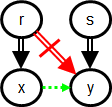Root-pair configuration 2

Here, the double-arrows represent the reachability (⇒) relation, not just parent-child relationships. The red double-arrow (with a stroke through it) means the complement of ⇒ (thus, in Configuration 1, s ⇏ x). It does not matter whether or not r ⇒ y (resp. s ⇒ x) in Configuration 1 (resp. Configuration 2). In other words, at least one of r ⇏ y and s ⇏ x must be the case. The dotted green arrow means that (x, y) ∈ (ssb − ⇒), in other words, that x precedes y without being an ancestor of it.

An instance of at least one of those configurations is found in each of the following CODGs:Example 7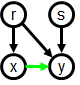Example 8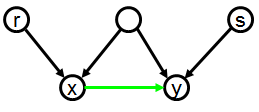Example 9

6.2 Lemma Let G be a CODG, and r and s be two roots in G such that r ↝ s. Then, for each H that is a CA sre of G, (r, s) ∈ gsp(H).

Proof sketch. If there exists no CA sre of G, or if there are no two roots r and s in G such that r ↝ s, then the lemma is vacuously verified. Let thus H be any CA sre of G and, for the sake of contradiction, suppose r and s are two G-roots such that r ↝ s and such that (r, s) ∉ gsp(H). Suppose x and y are two vertices as in Configuration 1 above (we prove only the case of Configuration 1; that of Configuration 2 is proved similarly).

By the definition of sre, if (r, s) ∉ gsp(H), then it must be the case that (s, r) ∈ gsp(H), and thus, by construction of ssb(H) (Definition 4.3-2), that (s, r) ∈ ssb(H). Hence, it follows by Lemma 4 of M2008 and the fact that r and s do not stand in ancestor-descendant relationship (being both G-roots, they are ⇒-incomparable), that (r, s) ∈ sea(H). Similarly, from (x, y) ∈ ssb(H) and x ⇏ y, and y ⇏ x (because (x, y) ∈ ssb(H)), we conclude that (y, x) ∈ sea(H).

Now, by construction of sea(H) (Definition 4.3-4), and from the facts that r ⇒ x, that (r, s) ∈ gsp-1(H), and that s ⇏ x, we conclude that (x, s) ∈ sea(H). So, we have (y, x) ∈ sea(H) (established earlier), and (x, s) ∈ sea(H), and (s, y) ∈ sea(H) (because s ⇒ y). Thus, sea(H) is cyclic, contrary to our hypothesis that H is CA, and so we must reject the hypothesis that (r, s) ∉ gsp(H), and conclude that (r, s) ∈ gsp(H).

QED

6.3 Theorem A CODG is FCA iff it is CA and it does not have any two roots r and s such that r ↝ s and s ↝ r.

Proof sketch.

(⇒) Any FCA CODG is CA. If a CODG had roots r and s such that r ↝ s and s ↝ r, then by Lemma 6.2, it would have a cycle in ssb, and thus could not be CA.

(⇐) Let G be a CA CODG in which no two roots r and s are such that r ↝ s and s ↝ r. Note that by Lemma 5.13 and the fact that G is CA, we know that G is sp-consistent and reduced, and will thus take this for granted.

We give an algorithm for constructing an ordering of the roots of G that can be used as the root-order in a sre H of G which will be shown to be FCA. In the algorithm, ssb(G, ROR) denotes the result of building ssb as per Definitions 4.3-1 and 4.3-2, but using gsp(G) ∪ ROR instead of gsp(G)

Algorithm:

1. Let ROR = { (r, s) | r and s are roots in G and r ↝ s }.

2. Let SSB = ssb(G, ROR).

3. WHILE (∃ x, y ∈ V)[ch(x) = ch(y) & {(x, y), (y, x)} ∩ SSB = ∅]

1. Pick any x and y satisfying the WHILE clause, and let X = x, and Y = y.

2. Let ROR = ROR ∪ {(r, s) | r and s are roots in G, and r ⇒* X, and s ⇒* Y}.

3. Let SSB = ssb(G, ROR).

When the algorithm stops, ROR is the root-order to be used for constructing H.

The intuition behind the algorithm is best conveyed with examples. Essentially, the algorithm goes like this: start with the root-orderings that are imposed by the topology of the CODG, i.e., those pairs of roots (r, s) for which r ↝ s. Then, for the other root-pairs, they can basically be ordered randomly, as long as no silly decision is taken.

To see what silly decisions would be, consider Example 10. A silly decision would be to stick root r between s and t. To avoid such decisions, the root-ordering must be built gradually, considering one by one (the order does not matter) the unordered pairs of parents of the same children. For each such pair (x, y), decide of an arbitrary order, then, make sure all roots reachable upwards from x come before all those reachable upwards from y (there can be no root reachable both ways, otherwise, x and y would have been ordered to start with).

Each time such root-orderings are added, the consequences on the global ordering of the CODG are recomputed and propagated down from the roots.

Example 11 provides a more intricate example, in which eight possible pairs of unordered parents of the same children exist: (u, w), (u, x), (v, w), and (v, x), and their inverse. Whichever pair is chosen, it will result in roots r and s being ordered at step 3b, and then, in all eight pairs being ordered at step 3c.

Examples 12 and 13 show that the addition of a leaf can cause some root-pairs to be ordered from the start: (r ↝ s) and (r ↝ t) in Example 12; (r ↝ s) in Example 13.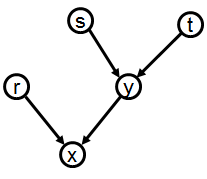Example 10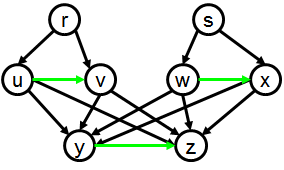Example 11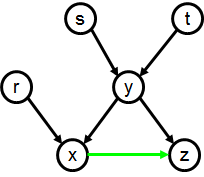Example 12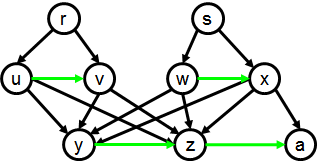Example 13

Proof sketch of termination: At each turn, at least one pair of the kind sought for in (3) is ordered. Indeed, it can be shown that the chosen pair (x, y) causes arcs to be added to ROR that will necessarily order the pair (x, y) itself. Thus, eventually, no pair satisfying the WHILE clause will remain.

Proof sketch that ROR orders all pairs of roots: The existence of an unordered root-pair implies that there is a pair satisfying the WHILE clause. Thus, when no more such pair exists, all the roots have been ordered.

Proof sketch that ssb(G, ROR) is acyclic: Any condition that might cause a cycle would also cause a cycle in { (r, s) | r and s are roots in G and r ↝ s }, a contradiction.

A rough analysis of the algorithm shows that its running time is polynomial (probably with degree at most 3 or 4). Obviously, it could be used to actually build a CA sre of any FCA CODG, and thus an oo-serialization of the CODG. This establishes a polynomial upper-bound on the task of verifying full-completion-acyclicity and of generating an oo-serialization of a FCA CODG. While interesting, we do not believe these upper-bounds to be tight, and hence consider the exact complexity of these tasks to be open questions.

## 7. Conclusion and future work

In this paper, we defined a class of graphs, child-arc-ordered directed graphs (CODGs), that includes multi-graphs and non-acyclic graphs, and investigated the conditions under which a CODG is “oo-serializable”, i.e., has a structure which corresponds to that of an overlap-only marked-up document. We found that the property of completion-acyclicity does not guarantee oo-serializability in general for CODGs, by showing that there exist completion-acyclic CODGs that are not oo-serializable. By contrast, Marcoux has shown that for the less general class of node-ordered-DAGs (noDAGs), completion-acyclicity does guarantee oo-serializability M2008.

We then defined a condition strictly stronger than completion-acyclicity, full-completion-acyclicity, and showed that it does guarantee oo-serializability for all CODGs.

Finally, we presented polynomial-time algorithms for checking full-completion-acyclicity and for computing an oo-serialization of fully-completion-acyclic CODGs. However, we do not believe these algorithms to be optimal. Thus, open questions include determining the exact complexity of — and finding optimal algorithms for — checking completion-acyclicity, full-completion-acyclicity, and of actually computing an oo-serialization of a CODG once it is found to be serializable.

Another area of research we hope to pursue in the near future is investigating whether and how some forms of interrupted and virtual elements, as found in TexMecs, can be characterized in terms of graphs.

## Appendix A. Notation and symbols

For the convenience of readers who find the notation used here unfamiliar, we list here the symbols and conventional variable names used in this paper.

 ↝ the must-precede relation on roots of a graph G: r ↝ s if and only if there exist vertices x and y standing in some specific configurations with respect to r and s (see Definition 6.1). ⇒G the positive transitive closure of E(G), for any graph G; sometimes known as the reachability relation of G; often abbreviated to ⇒ when the identity of G is understood. ⇒*G the reflexive transitive closure of E(G), for any graph G; often abbreviated to ⇒* when the identity of G is understood. ∈ is an element of. So x ∈ y means that x is an element in the set y. ∪ set union. So x ∪ y denotes the set of all elements which are members either of set x or of set y or both. ∩ set intersection. So x ∩ y denotes the set of all elements which are members of both set x and set y. ap(G) the ancestral-precedence relation of a graph G; abbreviated ap when G is understood. This is a binary relation consisting of all node pairs (v, w) for which (informally) v is an ancestor of w and not vice-versa. ch (core meaning) a unary function that maps each node of a graph to a sequence of nodes of that graph. (secondary meaning) a two-argument function that maps from a pair (v, n) (where v is a node and n is an integer) to at most one node among the children of v. ch(v) for any node v in a graph G, ch(v) denotes a sequence of nodes in G. Note that sequences are typically modeled as sets of pairs (n, e) where n is a number and e an element of the sequence. The set of pairs denoted by ch(v) can thus be treated as a function from non-negative integers to nodes in the graph: for any node v and any suitable integer n, ch(v)(n) denotes the nth child of v; ch(v)(0) denotes the first child, ch(v)(1) denotes the second, etc. To reduce the need for parentheses, ch(v)(n) is normally written in the simpler form ch(v,n). ch(v, n) denotes the nth child (counting from 0) of node v. This is a short-hand form for the expression ch(v)(n). E(G) the set of arcs in a graph G G the conventional variable for a graph (here invariably a CODG) gsp a shorthand form of gsp(G) when the identity of the graph G is understood. gsp(G) the global sibling-precedence relation of a graph G; written as sp when G is understood. This is (speaking informally) a relation consisting of all node pairs (v, w) which share a parent, and for which v precedes w among the children of that parent. Note that the same pair of children may share more than one parent, occurring in one order for one parent and in the other order for the other parent. So gsp(Example 2.3) includes both (b, c) and (c, b). N the natural numbers (0, 1, 2, ...) n, m conventional variables used to represent individual natural numbers r conventional variable used to denote an arbitrary range. r1 for a given range r, r1 denotes the first element of r. r2 for a given range r, r2 denotes the second element of r. r, s conventional variables used to denote two roots of a CODG. sea(G) the should-end-after relation of a graph G; abbreviated sea when G is understood seaa(G) the should-end-after additions relation of a graph G; abbreviated seaa when G is understood sp a shorthand form of sp(G) when the identity of the graph G is understood. sp(G) the sibling-precedence relation of a graph G; this is a ternary relation consisting of all node triples (v, w, x) for which (informally) w precedes x among the children of v; note that if w occurs more than once among the children of v, then the triple (v, w, w) appears in sp. If some node x occurs between the two occurrences of w, then (v, w, x) and (v, x, w) are both in sp. sre single-rooted extension of some graph G. Note that any multi-rooted graph G has many single-rooted extensions (one for each possible ordering of the roots of G). ssb(G) the should-start-before relation of a graph G; abbreviated ssb when G is understood ssba(G) the should-start-before additions relation of a graph G; abbreviated ssba when G is understood V the set of nodes (or vertices) in a graph; a short-hand for V(G) V(G) the set of vertices in a graph G V × V the Cartesian product of V, the set of nodes; that is, the set of pairs (v, w) where v ∈ V and w ∈ V V × V × V the set of triples (v, w, x) where v, w and x are all ∈ V. v, w, x, u variables conventionally used for individual nodes in a graph (by convention, all of v, w, x, u ∈ V)

## References

[B1995] David Barnard, Lou Burnard, Jean-Pierre Gaspart, Lynne A. Price, C. M. Sperberg-McQueen, and Giovanni Battista Varile. “Hierarchical Encoding of Text: Technical Problems and SGML Solutions”, in Computers and the Humanities, 29/3 1995, pp. 211-231. http://www.springerlink.com/content/p7775247276v88h3/, http://xml.coverpages.org/barnardHier-ps.gz. doi:https://doi.org/10.1007/BF01830617

[D2004] Steven DeRose. “Markup Overlap: A Review and a Horse”. Paper delivered at Extreme Markup Languages, 2004, Montréal. http://conferences.idealliance.org/extreme/html/2004/DeRose01/EML2004DeRose01.html

[HS2003] Claus Huitfeldt and C. M. Sperberg-McQueen. TexMECS: An experimental markup meta-language for complex documents. University of Bergen, January 2001, rev. October 2003. http://mlcd.blackmesatech.com/mlcd/2003/Papers/texmecs.html

[M2008] Yves Marcoux. Graph characterization of overlap-only TexMECS and other overlapping markup formalisms. Proceedings of the Balisage 2008 conference, 12-15 august 2008, Montréal (Canada). doi:https://doi.org/10.4242/BalisageVol1.Marcoux01

[M2012] Moore, Neil. Multihierarchical documents and fine-grained access control (2012). Theses and Dissertations--Computer Science. Paper 6. http://uknowledge.uky.edu/cs_etds/6

[SH2004] C. M. Sperberg-McQueen and Claus Huitfeldt. GODDAG: A Data Structure for Overlapping Hierarchies. Springer-Verlag (2004). Preprint at http://cmsmcq.com/2000/poddp2000.html

[SH1999] C. M. Sperberg-McQueen and Claus Huitfeldt: “Concurrent Document Hierarchies in MECS and SGML”, in Literary and Linguistic Computing, 14 1999, pp. 29-42. http://llc.oxfordjournals.org/cgi/content/abstract/14/1/29. doi:https://doi.org/10.1093/llc/14.1.29

[W2005] Andreas Witt. “Multiple Hierarchies: New Aspects of an Old Solution”, in: Stefanie Dipper, Michael Götze, and Manfred Stede (eds.), Heterogeneity in Focus: Creating and Using Linguistic Databases, vol. 2 of Interdisciplinary Studies on Information Structure (ISIS), Working Papers of the SFB 632. University of Potsdam, Germany, 2005. (Corrected reprint of an Extreme Markup 2004 paper.) http://www.sfb632.uni-potsdam.de/publications/isis02_4witt.pdf

 The authors thank Deborah A. Lapeyre and several anonymous Balisage peer reviewers for their extensive help in improving the presentation of this paper.

 We focus here exclusively on graphs as the abstract structures conveyed by marked up documents.

 A number of other equivalent encodings are of course possible. The `q` elements can be fragmented in TEI style to signal that multiple XML elements together make up a single logical unit. In a Goddag structure built from this example, one would expect to find one node in the graph for each logical `q` element, rather one for each `q` element in the XML.

```<div xmlns="http://www.tei-c.org/ns/1.0">
<p>
<verse n="Jer.2.1">Moreover the word
of the LORD came to me, saying,</verse>
<verse n="Jer.2.2">
<q xml:id="Q-Jer.2.2-A" part="I">
Go and cry in the hearing of
Jerusalem, saying,
<q xml:id="Q-Jer.2.2-B" part="I">
Thus says the LORD:
<q xml:id="Q-Jer.2.2-C" part="I">
I remember you,
The kindness of your youth,
The love of your betrothal,
When you went after Me in the wilderness,
In a land not sown.
</q>
</q>
</q>
</verse>
<verse n="Jer.2.3">
<q part="M" prev="#Q-Jer.2.2-A">
<q part="F" prev="#Q-Jer.2.2-B">
<q part="F" prev="#Q-Jer.2.2-C">
Israel [was] holiness to the LORD,
The firstfruits of His increase.
All that devour him will offend;
Disaster will come upon them,
</q>
says the LORD.
</q>
</q>
</verse>
</p>
</div>
```

Or the `verse` elements can be fragmented. Or TEI virtual elements can be used to represent parts of the document structure that do not fit neatly into a hierarchy.

 Readers who have not recently reviewed the definition of TexMecs may need to be reminded of some basics of TexMecs notation. The conventions used here are these; most are adopted from HS2003 but bilocation tags are new; they are introduced in order to allow the serialization of a larger class of graphs.

• Start-, end-, and sole-tags for an element of type `e` take the forms `<e|`, `|e>` `<e>`.

• Elements may overlap.

• A unique identifier may be assigned to an element by following its generic identifier immediately with `@` and an ID value. These IDs are recognized and handled at the TexMecs level; they do not require declaration or application-level semantics.

• The generic identifier may be omitted from start- and end-tags, in which case they mark a pseduo-element: an arbitrary portion of the document, typically marked this way in order to assign an identifier to that segment of the text. In the examples in this paper, we assume a convention that wrapping a text node in such an anonymous element does not create a new node but merely gives an identifier for the text node. (This is not stated normatively in the definition of TexMecs.)

• The notation `<^e^xyz>` marks a virtual element, whose type is `e` and whose children are those of the element whose ID is `xyz`. Virtual elements thus serve as additional parents to nodes already present with other parents.

The analogous notation `<^^xyz>` is used to refer to the pseudo-element whose ID is `xyz`.

• The notation `<=xyz=>` is a bilocation tag, used to signal that the element whose ID is `xyz` appears as a child of the immediately open elements, at the location indicated.

Bilocation tags are not defined in HS2003; they are defined here in order to have a convenient notation for the graphs in this paper. When a bilocation tag appears in a TexMecs document, all the same parent-child arcs are created in the document graph as would be created were there a sole-tag at that location. But the target of the parent-child arcs is not a new element represented by a sole tag, but the element whose ID appears in the bilocation tag.

The example given here could be represented without bilocation tags, by adopting the convention that neither pseudo-elements nor virtual-element references to pseudo-elements create new nodes during parsing. Verse 3 would look like this using this convention.

```      <[email protected]|
<^^Jer.2.3a>
says the LORD.
|verse>```

 This may sound like a fragile or error-prone convention, but it turns out to work well most of the time, and it makes the diagrams easier to read than attaching numbers to the arcs and drawing them with fewer crossings but out of order.

 Example 4 is oo-serializable in the TexMecs form ```<poem| <verse| <quote~1| leaves <quote~2| fall |verse> |quote~1> |quote~2> |poem>```.

Example 5 is not oo-serializable, in brief, because its EA (ends-after) relation has a cycle among the first `quote` element, the `verse` element, and the text node containing the word `fall`. Let us refer to them as q1, v, and f for short.

• q1 follows v among the children of the `poem` element, and thus q1 must end after v.

• v dominates f, and thus v must end after f.

• f is not dominated by q1, but it is dominated by a following sibling of q1 (namely, the second `quote` element), and thus f must end after q1.

It is the third constraint, not present in Example 4, which makes the difference between the oo-serializability of the two examples.

 The curious reader may, however, be interested in the enumeration. We cannot give it in full, but we can sketch it here.

In Example 6, nodes a and e are non-terminals (which means they must correspond to elements in TexMecs) and nodes b, c and d are childless (which means they may correspond either to empty elements or to spans of character data in TexMecs, that is, to leaf nodes). One way to begin the enumeration is to observe that in overlap-only TexMecs the elements a and e will each have one start- and one end-tag. There are six possible orders for these four tags:

1. `<a| <e| |a> |e>`

2. `<a| <e| |e> |a>`

3. `<a| |a> <e| |e>`

4. `<e| <a| |a> |e>`

5. `<e| <a| |e> |a>`

6. `<e| |e> <a| |a>`

Note that the possible number of orderings for any n items is n! (n factorial), so the total number of orderings for the four tags involved here is 24 (= 4!). Of those 24, half are ill-formed because in them the end-tag for a precedes its end-tag; of the remaining 12, half are ill-formed because the end-tag of e precedes the start-tag.

To each of these six patterns for the representation of nodes a and e there correspond 210 possible TexMecs documents with nodes b, c, and d interleaved among the tags for a and e. (Node b can be situated in any of five locations: before the first tag, after the first tag, after the second, after the third, after the fourth. The tag for node c can be placed in any of six locations (before or after any of the four start- and end-tags and node b); the tag for node d can be placed in any of seven locations. 210 = 5 × 6 × 7.) The beginning of the enumeration might look like this:

• d c b <a| <e| |a> |e>

• c d b <a| <e| |a> |e>

• c b d <a| <e| |a> |e>

• c b <a| d <e| |a> |e>

• c b <a| <e| d |a> |e>

• c b <a| <e| |a> d |e>

• c b <a| <e| |a> |e> d

• d b c <a| <e| |a> |e>

• b d c <a| <e| |a> |e>

• b c d <a| <e| |a> |e>

• b c <a| d <e| |a> |e>

• d b <a| c <e| |a> |e>

• b d <a| c <e| |a> |e>

• b <a| d c <e| |a> |e>

• b <a| c d <e| |a> |e>

• b <a| c <e| d |a> |e>

To each of these 210 interleavings of b, c, and d into the four start- and end-tags for a and e, there correspond eight TexMecs documents. Each of nodes b, c, and d may be either a text node or an empty element, so there are eight (two to the third power) combinations. The first pattern in the preceding list corresponds to the following eight TexMecs documents, and each of the other 210 expands similarly.

• d c b <a| <e| |a> |e>

• <d> c b <a| <e| |a> |e>

• d <c> b <a| <e| |a> |e>

• <d> <c> b <a| <e| |a> |e>

• d c <b> <a| <e| |a> |e>

• <d> c <b> <a| <e| |a> |e>

• d <c> <b> <a| <e| |a> |e>

• <d> <c> <b> <a| <e| |a> |e>

In total, then, there are 6 patterns for the start- and end-tags of a and e, 210 ways to interleave b, c, and d into those patterns, and 8 ways to realize each interleaving, for 10,080 (6 × 210 × 8) structurally possible oo-TexMecs documents for this configuration of five nodes. Since each of the five nodes can be either a leaf node or a non-terminal, there are 32 possible configurations. These vary in their number of possible realizations, but we hope it is now clear why enumerating the distinct documents for five-node graphs seems unlikely to be a helpful approach.

×

David Barnard, Lou Burnard, Jean-Pierre Gaspart, Lynne A. Price, C. M. Sperberg-McQueen, and Giovanni Battista Varile. “Hierarchical Encoding of Text: Technical Problems and SGML Solutions”, in Computers and the Humanities, 29/3 1995, pp. 211-231. http://www.springerlink.com/content/p7775247276v88h3/, http://xml.coverpages.org/barnardHier-ps.gz. doi:https://doi.org/10.1007/BF01830617

×

Steven DeRose. “Markup Overlap: A Review and a Horse”. Paper delivered at Extreme Markup Languages, 2004, Montréal. http://conferences.idealliance.org/extreme/html/2004/DeRose01/EML2004DeRose01.html

×

Claus Huitfeldt and C. M. Sperberg-McQueen. TexMECS: An experimental markup meta-language for complex documents. University of Bergen, January 2001, rev. October 2003. http://mlcd.blackmesatech.com/mlcd/2003/Papers/texmecs.html

×

Yves Marcoux. Graph characterization of overlap-only TexMECS and other overlapping markup formalisms. Proceedings of the Balisage 2008 conference, 12-15 august 2008, Montréal (Canada). doi:https://doi.org/10.4242/BalisageVol1.Marcoux01

×

Moore, Neil. Multihierarchical documents and fine-grained access control (2012). Theses and Dissertations--Computer Science. Paper 6. http://uknowledge.uky.edu/cs_etds/6

×

C. M. Sperberg-McQueen and Claus Huitfeldt. GODDAG: A Data Structure for Overlapping Hierarchies. Springer-Verlag (2004). Preprint at http://cmsmcq.com/2000/poddp2000.html

×

C. M. Sperberg-McQueen and Claus Huitfeldt: “Concurrent Document Hierarchies in MECS and SGML”, in Literary and Linguistic Computing, 14 1999, pp. 29-42. http://llc.oxfordjournals.org/cgi/content/abstract/14/1/29. doi:https://doi.org/10.1093/llc/14.1.29

×

Andreas Witt. “Multiple Hierarchies: New Aspects of an Old Solution”, in: Stefanie Dipper, Michael Götze, and Manfred Stede (eds.), Heterogeneity in Focus: Creating and Using Linguistic Databases, vol. 2 of Interdisciplinary Studies on Information Structure (ISIS), Working Papers of the SFB 632. University of Potsdam, Germany, 2005. (Corrected reprint of an Extreme Markup 2004 paper.) http://www.sfb632.uni-potsdam.de/publications/isis02_4witt.pdf